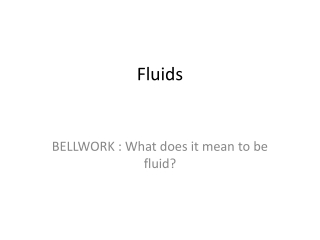DownloadDownload PresentationFluids

# Fluids

Télécharger la présentation## Fluids

- - - - - - - - - - - - - - - - - - - - - - - - - - - E N D - - - - - - - - - - - - - - - - - - - - - - - - - - -
##### Presentation Transcript

1. Fluids BELLWORK : What does it mean to be fluid?

2. Bellwork – 01/24/17 • You are on an ice-covered pond and the ice starts to crack – what do you do?

3. Bellwork – 02/01/16 • On ice and it starts to crack – what do you do? • Lay down flat • Distributes weight over more surface area

4. BELLWORK - Pressure • What is pressure?

5. TN Standards CLE.3202.1.8 – Investigate relationships among the pressure, temperature, and volume of gases / liquids CLE.3202.Inq.3 – Use appropriate tools and technology to collect precise and accurate data CLE.3202.Inq.6 – Communicate and defend scientific findings

6. Pressure [ Chpt 3 – section 3 ] • What is pressure? • Feeling forced to do something • A force needed to squash an object • Force exerted per unit area of contact

7. PhET simulation • Heat water to steam ( turn heat up at bottom ) • What happened to pressure in a closed container? • So why does the container ‘splode??

8. Balloons • While it is running, we’ll use balloons as an example of pressure • How does amount of gas in balloon relate to its size?

9. Balloons • What direction is the gas inside the balloon pointing to?

10. Balloons • What direction is the gas inside the balloon pointing to if really hot?

11. Balloons • What can we say about the pressure found in balloons of different sizes? ( start out the same size ) • Small one • Big one

12. Balloons • What can we say about the pressure found in balloons of different sizes? ( start out the same size ) • Small one • Big one PRESSURE IS LARGER IN THE BIGGER ONE

13. Challenge • What is a possible mathematical relationship for pressure? • Force and surface area are involved • What are independent and dependent variables?

14. Pressure • Force exerted per unit area of a surface • Pressure = F / A ( force divided by area )

15. Pressure - Example • Write down or Estimate your weight in lbs : • Estimate the area of your shoe-sole ( in2 ): • x “ wide & y “ long • Shoe area = • Pressure when standing on floor equals: • Weight / total shoe-area

16. Pressure - Example • Write down or Estimate your weight in lbs : • 190 lbs • Estimate the area of your shoe-sole ( in2 ): • Mine – 4.25 “ wide & 12 “ long • Shoe area = 51 x 2 = 102 in2 • Pressure when standing on floor equals: • Weight / total shoe-area • 1.9 psi exerted on floor

17. Pressure – Math Relationships • Force is directly proportional and surface area of ( contact ) is inversely proportional to pressure • P = F / A • Increase force  increase pressure • Increase area  DECREASE pressure

18. Buoyant Force • A buoyant force pushes the boat up • All fluids exert an upward buoyant force on matter

19. Buoyant Force • Archimedes’ principle states that an object floats if the buoyant force on the object is equal to the object’s weight • An object’s ability to float is based on the buoyant force, density of the fluid, and the density of the object

20. Hydrostatic Pressure • Pressure felt by a column of fluid ( so, just being under a certain amount of fluid ) • Does not matter how much volume of fluid there is – ONLY the depth • Pressure = fluid density * gravity * depth

21. Pascal’s Principle • An enclosed fluid exerts pressure equally in all directions • A change in the external pressure exerted on an enclosed fluid is transmitted equally and unchanged in all directions ( every point ) within that fluid

22. Work with Partners • Study Guides • Pg 12 • #2, 3 ( put in N / cm2)

23. Challenge / Exit Pass • Consider a submarine at the bottom of the ocean. • What is the water doing to the submarine • Why does the submarine not get crushed?

24. PLC Day / Upcoming in Week • Gonna watch stuff ‘splode ?? • Open-Notes Quiz Thursday/Friday

25. Exit Pass